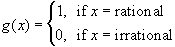#Interactive Real Analysis

Next | Previous | Glossary | Map

## 6.3. Discontinuous Functions

### Examples 6.3.4(d):

What kind of discontinuity does the function g(x) have at every point (with proof).This function is impossible to graph. The picture above is only a poor representation of the true graph. Nonetheless, take an arbitrary point x0 on the real axis. We can find a sequence {xn} of rational points that converge to x0 from the right. Then g(xn) converges to 1. But we can also find a sequence {xn} of irrational points converging to x0 from the right. In that case g(xn) converges to 0. But that means that the limit of g(x) as x approaches x0 from the right does not exist. The same argument, of course, works to show that the limit of g(x) as x approaches x0 from the left does not exist. Hence, x0 is an essential discontinuity for g(x).
Next | Previous | Glossary | Map